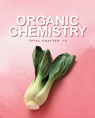×#### Thank you for registering.

One of our academic counsellors will contact you within 1 working day.

Click to Chat

1800-1023-196

+91-120-4616500

CART 0

• 0

MY CART (5)

Use Coupon: CART20 and get 20% off on all online Study Material

ITEM
DETAILS
MRP
DISCOUNT
FINAL PRICE
Total Price: Rs.

There are no items in this cart.
Continue Shopping
```
How to determine the rate determination step(i.e slow step) in any process. In case when activation energy is given and in case when Ea is not given

```
11 years ago

```							Hi
If activation energy is not given then u need to consider the mechanism, the reaction which is most hindered and is difficult to occur is the slowest and hence the rate determining step . This can be predicted by applying the various concepts like steric hindrance, stabilisation of products by h bonding etc and hence the slowest rxn can be determined.
one of the property of mechanisms is that they must predict the experimentally determined rate law.  To calculate the rate law from a mechanism you need to first know the rate limiting step. The rate limiting step determines the rate of the reaction because it is the slowest step.
Activation Energy and the Arrhenius Equation

Intuitively, it makes sense that a reaction with a higher activation barrier will be slower.  Think of how much harder you must roll a ball up a large hill than a smaller one. Let's consider chemical reactions more deeply to derive an equation which describes the relationship between the rate constant of a reaction and its activation barrier.  To simplify our derivation, we will assume that the reaction has a one-step mechanism.  This elementary step represents a collision as shown in .  Therefore, the frequency of the collisions, f,  will be important in our equation. Notice that only a certain orientation of the molecules will lead to a reaction.  For example, the following collision will not lead to a reaction.  The reagent molecules simply bounce off of one another:Figure 1.6: Only specific orientations during a collision will lead to a reaction.

Therefore, we will need to include an orientation factor (or steric factor),  p, that takes into account the fact that only a certain fraction of collisions will lead to  reaction due to the orientation of the molecules.  Another factor we must consider is that only a certain fraction of the molecules colliding will have enough energy to overcome the activation barrier.  The Boltzmann distribution is a thermodynamic equation that tells us what fraction of the molecules have a certain amount of energy.  As you know, at higher temperatures the average kinetic energy of the molecules increases.  Therefore, at higher temperatures more molecules have an energy greater than the activation energy--as shown in .Figure 1.7: Boltzmann distributions for T1 greater than T2

Combining the above considerations, we state the following relationship between the rate constant and the activation energy, called the Arrhenius equation:The variable k is the rate constant, which is dependent on the frequency of the  collisions f, orientation factor p, activation energy  Ea, and temperature T.  From the expression for the Arrhenius  equation you should note that a small increase in activation energy leads to a large decrease in rate constant.  Furthermore, temperature has a similarly exponential effect on the rate constant.  An experimental rule of thumb is that a 10oC increase in temperature leads to a doubling of the rate constant.
```
11 years ago
Think You Can Provide A Better Answer ?

## Other Related Questions on Organic Chemistry

View all Questions »### Course Features

• 731 Video Lectures
• Revision Notes
• Previous Year Papers
• Mind Map
• Study Planner
• NCERT Solutions
• Discussion Forum
• Test paper with Video Solution### Course Features

• 70 Video Lectures
• Revision Notes
• Test paper with Video Solution
• Mind Map
• Study Planner
• NCERT Solutions
• Discussion Forum
• Previous Year Exam Questions